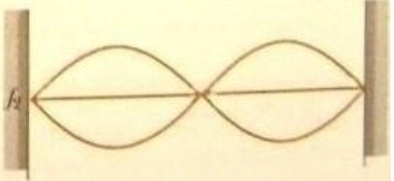# Problem: a) Suppose the tension is quadrupled while the frequency shaking the string is held constant. Will there be a standing wave? If so, draw it. If not, why not?b) Suppose the tension is tripled while the frequency shaking the string is held constant. Will there be a standing wave? If so how many antitodes will it have? If not, why not?

###### FREE Expert Solution

a)

From the diagram, the frequency, f:

$\overline{){\mathbf{f}}{\mathbf{=}}\frac{\mathbf{n}}{\mathbf{2}\mathbf{L}}\sqrt{\frac{\mathbf{T}}{\mathbf{\mu }}}}$

n is the number of loops, in this case, 2.

The velocity of the wave, v:

$\overline{){\mathbf{v}}{\mathbf{=}}\sqrt{\frac{\mathbf{T}}{\mathbf{\mu }}}}$###### Problem Detailsa) Suppose the tension is quadrupled while the frequency shaking the string is held constant. Will there be a standing wave? If so, draw it. If not, why not?

b) Suppose the tension is tripled while the frequency shaking the string is held constant. Will there be a standing wave? If so how many antitodes will it have? If not, why not?